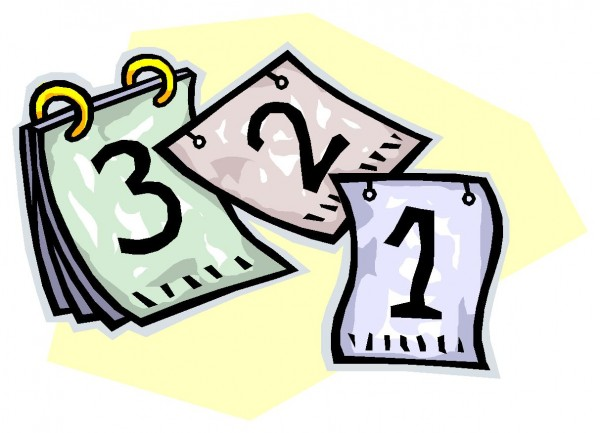Home Year

# Year

1826
0
SHAREThe derivatives of the word “year” (sinet, sinin) are mentioned 19 times in the Quran. The solar and lunar calendars need correction because of the leap year. When the world revolves around the sun 365 times, the moon revolves around the earth and itself 12 times.

This constitudes a year. But when the earth completes its revolvation and arrives at the starting point, the moon is behind the schedule. It takes the earth and the moon 19 years to meet at the same starting point. This cycle of 19 years is called the Meton cycle. The lunar calendar re-arranged in every 19 years, ends up with 7 leap years (355 days) and 12 full years (354 days) during this period.

It is also another surprising characteristic of the miracles that the singular year (sinet) is used 7 times, whereas the plural year (sinin) is used 12 times in the Quran. And all the derivatives of the word “year” are used 19 times, an indication of the Meton cycle.

 The word Number of occurrence The word Year 19
 In how many years a meton cycle occurs? 19

The earth lines up with the sun and the moon every 19 years. In the Quran, the words “sun” and “moon” are often used in the same verses, exactly 19 times; the same number that makes a “Meton cycle” corresponding to the same number the word “year” is used in the Quran. It is interesting to note that on the 19th time, these two words are used together, we have the following verse:

9- And the sun and the moon are conjoined.
75. The Resurrection, 9

The overlapping of the meaning and of the mathematical concordance is a miracle. Now let us look at the table where the words “sun” and “moon” are used together (In 41st sura, verse 37; these words are used twice).

 Serial no Sura Verse 1 6 96 2 7 54 3 10 5 4 12 4 5 13 2 6 14 33 7 16 12 8 21 33 9 22 18 10 29 61 11 31 29 12 35 13 13 36 40 14 39 5 15 41 37 16 41 37 17 55 5 18 71 16 19 75 9

If you add up the numbers of the suras and the verses these words are used:
6 + 96 + 7 + 54 + 10 + 5 + 12 + 4 + 13 + 2 + 14 + 33 + 16 + 12 + 21 + 33 + 22 + 18 + 29 + 61 + 31 + 29 + 35 + 13 + 36 + 40 + 39 + 5 + 41 + 37 + 41 + 37 + 55 + 5 + 71 + 16 + 75 + 9 = 1083 (the number 19X57) and 57=the number 19*3.

Please keep in mind this concordance formed by the multiples of 19, and remember it, in the following chapters on the miraculous number of 19.

The MMLC in the Quran shows itself both in the sura and verse numbers. This proves that the Quran’s miraculous order covers even the numbers of the suras and verses.